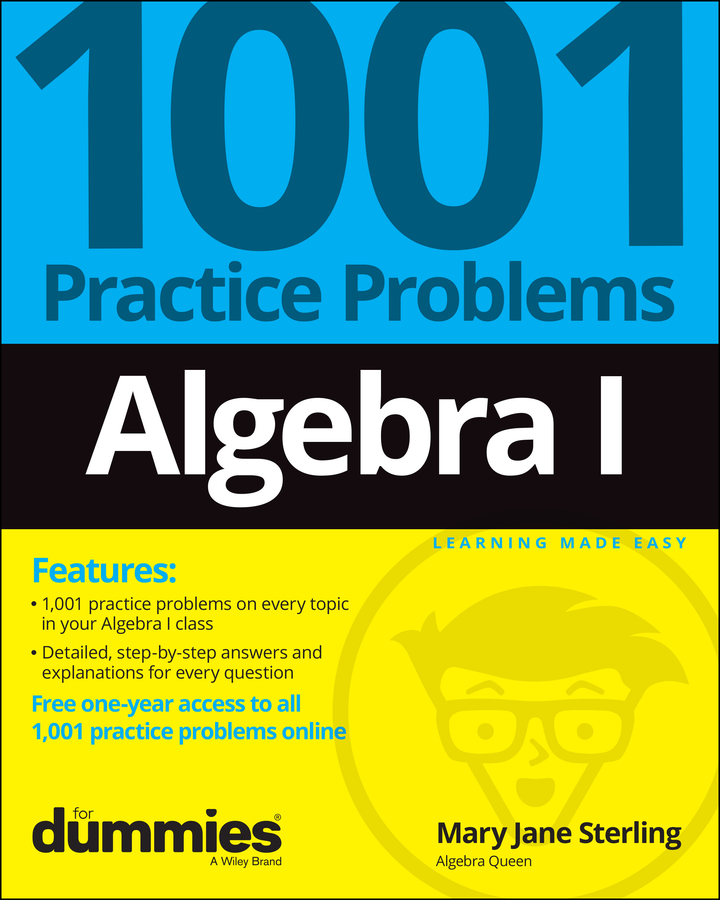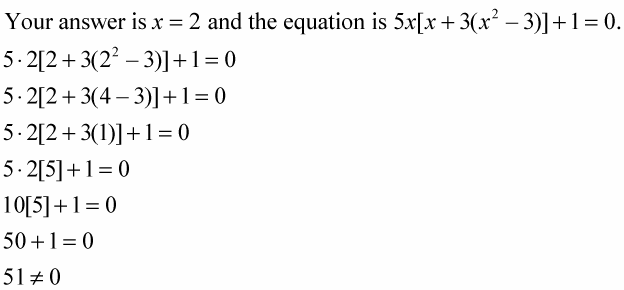##### Algebra I: 1001 Practice Problems For Dummies (+ Free Online Practice)Checking your answers when doing algebra problems is always a good idea — after all, if there's a way to ensure that you have the correct answer, it's worth the time, isn't it? You check your answers in algebra on two levels: first, you see if the answer actually makes sense, and then you plug your answer back into the problem to see if it works.

• Check to see whether the answer makes sense.

This first level of checking your answer weeds out the solutions that are obviously wrong — this is your "common sense" check. For instance, if your answer to an algebra problem is x = 5, and you were solving for Jon’s weight in pounds, you probably want to redo the work because five pounds doesn’t make any sense unless Jon is a guinea pig. On the other hand, if the problem involves a number of pennies in a person’s pocket, then five pennies seems reasonable.

• Plug the answer back into the problem to see if it works.

This second level of checking your answer is more critical because it gives you more exact information about your answer. Actually plugging in your answer requires you to go through the algebra manipulations in the problem. You add, subtract, multiply, and divide to see if you get a true statement using your answer.

For example, you need to perform the operations in the following equation after plugging in your answer for the variable x.

Your answer is x = 5 and the equation is x + (x + 4) = 14.

5 + (5 + 4) = 14

5 + (9) = 14

14 = 14